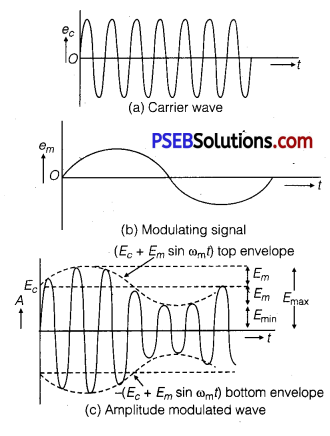# PSEB 12th Class Physics Important Questions Chapter 15 Communication Systems

Punjab State Board PSEB 12th Class Physics Important Questions Chapter 15 Communication Systems Important Questions and Answers.

## PSEB 12th Class Physics Important Questions Chapter 15 Communication Systems

Question 1.
Name the essential components of a communication system.
Transmitter, medium or channel, and receiver.

Question 2.
What is the function of a transducer used in a communication system?
Transducer used as a sensor or detector in communication system. It converts the physical signal into electrical signal.

Question 3.
What is the function of a repeater in a communication system?
Repeater pick up the signals from the transmitter, amplifies it and transmits it to the receiver. Thus, repeater comprises up of receiver, transmitted and amplifier. Its function is to extend the range of communication.

Question 4.
Define bandwidth and describe briefly its importance in communicating signals.
It is defied as the frequency range over which given equipment operates.
Importance: To design the equipment used in communication system for distinguishing different message signals.

Question 5.
Which basic mode of communication is used for telephonic communication?
Point to point is a basic mode of communication, which is used for telephonic conversation. In this mode of communication, communication takes place over a link between a single transmitter and a receiver.Question 6.
How are microwaves produced?
A type of electromagnetic wave is microwave whose wavelength ranging from as long as metre to as short as millimeter and having the frequency range 3000 MHz to 300 GHz. This also includes UHF, EHF and various sources with different boundaries.

Question 7.
What is sky wave propagation?
Skywave propagation is a mode of propagation in which communication of radiowaves in frequency range 2 MHz-20 MHz takes place due to reflection from the ionosphere.

Question 8.
Would sky waves be suitable for transmission of TV signals of 60 MHz frequency? (NCERT Exemplar)
A signal to be transmitted through sky waves must have a frequency range of 1710 kHz to 40 MHz. But, here the frequency of TV signals are 60 MHz which is beyond the required range. So, sky waves will not be suitable for transmission of TV signals of 60 MHz frequency.

Question 9.
How are sidebands produced?
Sidebands are produced due to the superposition of carrier wave of frequency ωc over modulating or audio signal of frequency ωm. The frequency of lower sideband is ωcm and the upper side band is ωcm

Question 10.
Why are broadcast frequencies (carrier waves) sufficiently spaced in amplitude modulated wave?
To avoid mixing up of signals from different transmitters. This can be done by modulating the signals on high-frequency carrier waves, e.g., frequency band for satellite communication is 5.925 – 6.425 GHz.

Question 11.
Why is the amplitude of modulating signal kept less than the amplitude of carrier wave?
The amplitude of modulating signal is kept less than the amplitude of carrier wave to avoid distortion.

Question 12.
What is the function of a bandpass filter used in a modulator for obtaining AM signal?
Bandpass filter rejects DC and sinusoid of frequency ωm, 2ωm, and 2ωc and retains frequencies ωc + ωm.
Thus, it allows only the desired frequencies to pass through it.

Question 13.
On radiating (sending out) and AM modulated signal, the total radiated power is due to energy carried by ωc, ωcm and ωcm. Suggest ways to minimise cost of radiation without compromising on information. (NCERT Exemplar)
In amplitude modulated signals, only sideband frequencies contain information.
Thus only (ωcm) and (ωc – ωm) contain information.

Now, according to question, the total radiated power is due to energy carried by
ωc, (ωc – ωm) and (ωcm)
Thus to minimize the cost of radiation without compromising on information, ωc can be left and transmitting
cm), (ωc – ωm) or both (ωcm) and (ωc – ωm).Question 14.
Two waves A and B of frequencies %MHz and 3 MHz, respectively are beamed in the sartie direction for communication via skywave. Which one of these is likely to travel longer distance in the ionosphere before suffering total internal reflection? (NCERT Exemplar)
As the frequency of wave B is more than wave A, it means the refractive index of wave B is more than refractive index of wave A (as refractive index increases with frequency increases). For higher frequency wave (i.e., higher refractive index) the angle of refraction is less i.e., bending is less. So, wave B travels longer distance in the ionosphere before suffering total internal reflection.

Question 1.
Draw a block diagram of a generalized communication system. Write the functions of each of the following:
(a) Transmitter
(b) Channel
The block diagram of a generalized communication system is shown in figureFunctions are as follows :
(a) Transmitter: It comprises of message signal source, modulator and transmitting antenna. Transmitter makes signals compatible for communication channel via modulator and antenna.
(b) Channel: It is a link for propagating the signal from transmitter to receiver.
(c) Receiver: It recovers the desired original message signals from the received signals at the end of channel.

Question 2.
Explain the terms
(i) Attenuation and
(ii) Demodulation used in communication system.
Attenuation: The loss in strength of a signal while propagating through a medium is known as attenuation.
Demodulation: The process of retrieval of information, from the carrier wave at the receiver end. This is the reverse process of modulation.

Question 3.
(a) Distinguish between ‘Analog and Digital signals’.
(b) Explain briefly two commonly used applications of the Internet.
(a) A signal that varies continuously with time (e. g., sine waveform) is called an analog signal.
A signal that is discrete is called a digital signal. The presence of signal is denoted by digit 1 and absence is denoted by digit 0.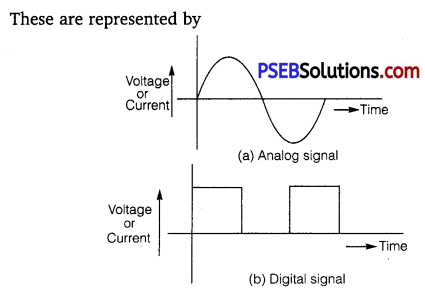(b) Uses of Internet: email, e-banking, e-shopping, e-ticketing, charting, surfing, file transfer, etc.

Question 4.
What is ground wave communication? Explain why this mode cannot be used for long-distance communication using high frequencies.
The mode of wave propagation in which wave guided along the surface of the earth is called ground wave communication.
The maximum range of propagation in this mode depends on
(i) transmitted power and
(ii) frequency (less than a few MHz)
At high frequencies, the rate of energy dissipation of the signal increases and the signal gets attenuated over a short distance.Question 5.
Define the term modulation. Draw a block diagram of a simple modulator for obtaining AM signals.
Or
Draw a block diagram of a simple modulator to explain how the AM wave is produced. Can the modulated signal be transmitted as such? Explain.
Modulation is the process in which low-frequency message signal is superimposed on high-frequency carrier wave so that they can be transmitted over long distances. The block diagram for a simple modulator for obtaining AM signal is shown as below :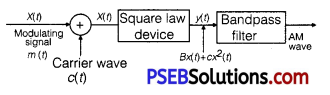Question 6.
Define modulation index. Why is it kept low? What is the role of a bandpass filter? Give its physical significance.
Modulation index is the ratio of the amplitude of modulating signal to that of carrier wave. Mathematically,
µ = $$\frac{A_{m}}{A_{c}}$$
Reason: It is kept low to avoid distortion.
Role: A bandpass filter rejects low and high frequencies and allows a band of desired frequencies to pass through it.
Physical Significance: It signifies the level of distortion or noise. A lower value of modulation index indicates a lower distortion in the transmitted signal.

Question 7.
State the concept of mobile telephony and explain its working.
Concept of mobile telephony is to divide the service area into a suitable number of cells centered on an office MTSO (Mobile Telephone Switching Office). Mobile telephony means that you can talk to any person from anywhere.
Explanation:

• Entire service area is divided into smaller parts called cells or cell zones.
• Each cell has a base station to receive and send signals to all the mobile phones present inside that cell.
• Each base station is linked to MTSO. MTSO coordinates between base station and TCO (Telephone Control Office).

Question 8.
What is space wave propagation? State the factors which limit its range of propagation. Derive an expression for the maximum line of sight distance between two antennas for space wave propagation.
Space Wave Propagation
The mode of propagation in which radio waves travel, along a straight line, from the transmitting to the receiving antenna.
Limiting Factors
(i) Curvature of the earth
(ii) Insufficient height of the receiving antenna
(iii) LOS distance (> 40 MHz) travel in straight line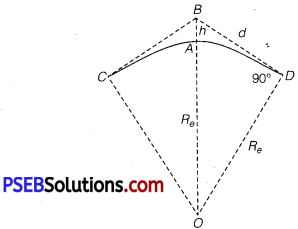Derivation : In right angled triangle BOD, ∠BDO =90°
∴BO2 = (OD)2 +(BD)2
le., (Re+h)2 =Re2 +(BD)2 ………………. (1)
As height h of the tower is very small as compared to radius (Re) of earth the point B will be very close to A, so that
∴ Equation (1) given (Re + h)2 = Re2 + d2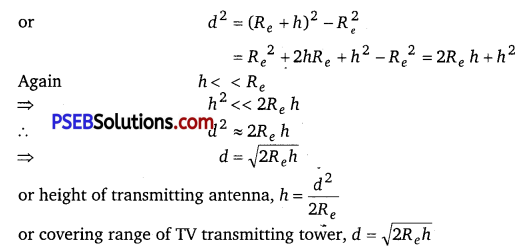or height of transmitting antenna, h =$$\frac{d^{2}}{2 R_{e}}$$
or covering range of TV transmitting tower, d = $$\sqrt{2 R_{e} h}$$
Thus, covering range of TV signal can be increased by increasing the height of transmission antenna.
For a transmitting antenna of height hT, and a receiving antenna of height hR, the maximum line of sight distance
becomes
dM= $$\sqrt{2 R h_{T}}+\sqrt{2 R h_{R}}$$

Question 9.
(a) Explain any two factors which justify the need of modulating a low-frequency signal.
(b) Write two advantages of frequency modulation over amplitude modulation
(a) (i) If λ is the wavelength of the signal then the antenna should have a length at least $$\frac{\lambda}{4}$$
For an electromagnetic wave of frequency 20 kHz, the wavelength λ is 15 km. Such a long antenna is not possible to construct and operate. So, there is need to modulate the wave in order to reduce the height of antenna to a reasonable height.

(ii) The power radiated by a linear antenna (length l) is proportional to $$\left(\frac{l}{\lambda}\right)^{2}$$.
This shows that power radiated increases with decreasing λ. So, for effective power radiation by antenna, there is need to modulate the wave.
(b)

• High frequency
• Less noise
• Maximum use of transmitted power

Question 10.
Given reasons for the following :
(i) For ground wave transmission, size of antenna (l) should the comparable to wavelength (λ) of signal, i.e. I = $$\frac{\lambda}{4}$$
(ii) Audio signals converted into an electromagnetic wave are not directly transmitted.
(iii) The amplitude of a modulating signal is kept less than the amplitude of carrier wave.
(i) To radiate the signals with high efficiency.
(ii) Because they are of large wavelength and power radiated by antenna is very small as
P ∝ $$1 / \lambda^{4}$$
(iii) It is so to avoid making over modulated carrier wave. In that situation, the negative half cycle of the modulating signal is dipped and distortion occurs in reception.Question 11.
Which of the following would produce analog signals and which would produce digital signals?(NCERT Exemplar)
(a) A vibrating tuning fork
(b) Musical sound due to a vibrating sitar string
(c) Light pulse
(d) Output of NAND gate
Analog and digital signals are used to transmit information, usually through electric signals. In both these technologies, the information such as any audio or video is transformed into electric signals. The difference between analog and digital technologies is that in analog technology, information is translated into electric pulses of varying amplitude. In digital technology, translation of information is into binary formal (zero or one) where each bit is representative of two distinct 4 amplitudes. Thus, (a) and (b) would produce analog signals and (c) and (d) would produce digital signals.

Question 12.
Why is AM signal likely to be more noisy than a FM signal upon transmission through a channel? (NCERT Exemplar)
In case of AM, the instantaneous voltage of carrier waves is varied by the modulating wave voltage. So, during the transmission, noise signals can also be added and receiver assumes noise a part of the modulating signal.
In case of FM, the frequency of carrier waves is changed as the change in the instantaneous voltage of modulating waves. This can be done by mixing and not while the signal is transmitting in channel. So, noise does not affect FM signal.

Question 1.
What does the term LOS communication mean? Name the types of waves that are used for this communication. What is the range of their frequencies? Give typical, examples, with the help of suitable figure of communication systems that use space wave mode propagation.
LOS Communication: It means “Line of sight communication”. Space wave are used for LOS communication.
In this communication, the space waves (radio or microwaves) travel directly from transmitting antenna to receiving antenna. Frequency for LOS communication must be more than 40 MHz.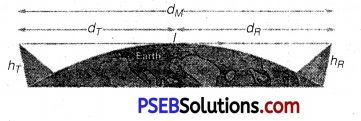If transmitting antenna and receiving antenna have heights hT and hR respectively, then radio horizon of transmitting antenna.
dT = $$\sqrt{2 R_{e} h_{T}}$$
where Re is radius of earth and radius horizon of receiving antenna,
dR = $$\sqrt{2 R_{e} h_{R}}$$
∴ Maximum line of sight distance,
dM = dT + dR = $$\sqrt{2 R_{e} h_{T}}+\sqrt{2 R_{e} h_{R}}$$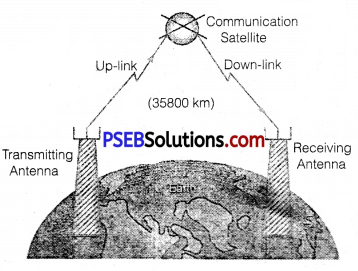The satellite communication is shown in figure. The space wave used is microwave.

Question 2.
(a) Distinguish between sinusoidal and pulse-shaped signals,
(b) Explain, showing graphically, how a sinusoidal carrier wave is superimposed on a modulating signal to obtain the resultant amplitude modulated (AM) wave.
(a) In the process of modulation, some specific characteristics of the carrier wave is varied in accordance with the information or message signal. The carrier wave maybe
(i) Continuous (sinusoidal) wave, or
(ii) Pulse, which is discontinuous.
A continuous sinusoidal carrier wave can be expressed as,
E = Eo sin (ωt +Φ)
Three distinct characteristics of such a wave are amplitude (E0), angular frequency (ω) and phase angle fcj)).
Any one of these three characteristics can be varied in accordance with the modulating baseband (AF) signal, giving rise to the respective Amplitude Modulation;
Frequency Modulation and Phase Modulation.

Again, the significant characteristics of a pulse are Pulse Amplitude, Pulse Duration or Pulse Width and Pulse Position (representing the time of rise or fall of the pulse amplitude). Any one of these characteristics can be varied in accordance with the modulating baseband (AF) signal, giving rise to the respective. Pulse Amplitude
Modulation (PAM), Pulse Duration Modulation (PDM), Pulse Width Modulation (PWM) and Pulse Position Modulation (PPM).

(b) Amplitude Modulation: When a modulating AF wave is superimposed on a high-frequency carrier wave in a manner that the frequency of modulated wave is same as that of the carrier wave, but its amplitude is made proportional to the instantaneous amplitude of the audio, frequency modulating voltage, the process is called amplitude modulation (AM).Let the instantaneous carrier voltage (ec) and modulating voltage (em) be represented by,
ec = Ec sinωc t …………………… (1)
em = Em sinωmt …………………….. (2)
Thus, in amplitude modulation, amplitude A of modulated wave is made proportional to the instantaneous modulating voltage em i.e.,
A = Ec+kem ……………………….. (3)
where k is a constant of proportionality.
In amplitude modulation, the proportionality constant k is made equal to unity. Therefore, maximum positive amplitude of AM wave is given
by.
A = Ec +em =Ec +Em sinωm t …………………….. (4)
It is called top envelope.
The maximum negative amplitude of AM wave is given by,
-A = – Ec – em
= – (Ec +Em sinωm t) …………………………. (5)